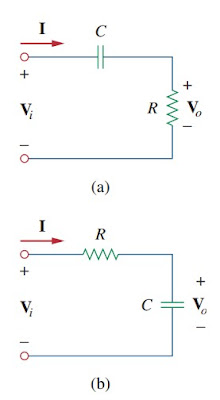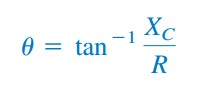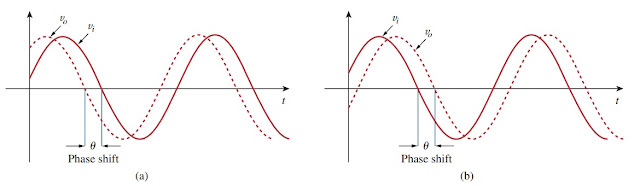omG8HI9LSKFW6lhCj8prTs0Z6lUhdOT9Jhi1Sf4m

# How to Calculate Phase Shifter Formula and Operation

The RC, RL, and RLC circuits have several ac applications; such as : coupling circuits, phase-shifting circuits, filters, resonant circuits, ac bridge circuits, and transformers. Among those applications, the simple application are RC phase-shifting circuits and ac bridge circuits. Phase shift operation principle is based on phasor.
Now we will learn about phase-shifting circuits.

## Phase Shifter

A phase-shifting circuit is often used to correct an undesirable phase shift which presents in a circuit or to produced special needed effect. An RC circuit is capable for this purpose because the capacitor causes the circuit current to lead the applied voltage. The two common examples can be seen in Figure.(1). (RL circuits or any reactive circuits could also serve the same purpose.)Figure 1. Series RC shift circuits : (a) leading output, (b) lagging output
In Figure.(1a), the circuit current I leads the applied voltage Vi by some phase angle θ, where 0 < θ < 90o, depending on the values of R and C. If XC = -1/ωC, then the total impedance is Z = R + jXC, and the phase shift is given by(1)
Notice that the phase shift amount is depended on the values of R, C, and the operating frequency. Since the output voltage Vo across the resistor is in phase with the current, Vo leads (positive phase shift) Vi as shown in Figure.(2a).Figure 2. Phase shift in RC circuits : (a) leading output, (b) lagging output
In Figure.(1b), the output is taken across the capacitor. The current I leads the input voltage Vi by θ, but the output voltage vo(t) across the capacitor lags (negative phase shift) the input voltage vi(t) as drawn in Figure.(2b).

We have to keep in mind that the simple RC circuits in Figure.(1) also act as voltage divider. Therefore, as the phase shift θ approaches 90o, the output voltage Vo approaches zero. For this reason, these simple RC circuits are used only when small amounts phase shift are required.

If it is desired to have phase shifts greater than 60o, simple RC networks are cascaded, thereby providing a total phase shift equal to the sum of the individual phase shifts. In practice, the phase shifts due to the stages are not equal, because the succeeding stages load down the earlier stages unless op amps are used to separate the stages.

## Phase Shifter Examples

For better understanding let us review examples below :
Solution :
If we select circuit components of equal ohmic value, say R = |XC| = 20 Ω, at a particular frequency, according to Equation.(1), the phase shift is exactly 45o. By cascading two similar RC circuits in Figure.(1a), we obtain the circuit in Figure.(3),Figure 3
providing a positive or leading phase shift of 90o, as we shall soon show. Using the series-parallel combination technique, Z in Figure.(3) is obtained as(1.3)
Substituting Equations(1.2) into (1.3) results
Hence, the output leads the input by 90o but its magnitude is only about 33% of the input.

2. For the RL circuit shown in Figure.(4a), calculate the amount of phase shift produced at 2 kHz.
At 2 kHz, we transform the 10 mH and 5 mH inductances to the corresponding impedances.
Consider the circuit in Figure.(4b). The impedance Z is the parallel combination of j125.7 Ω and 100 + 62.83 Ω. Thus,
showing that the output is about 19% of the input in magnitude but leading the input by 100o. If the circuit is terminated by a load, the load will affect the phase shift.

Untuk Bahasa Indonesia baca Rangkaian Penggeser Fasa Rangkaian AC.
Related Posts
SHARE